Next: Fourier Decomposition Optimization Up: Optimization - General Bound Previous: General Bound Maximization and

# Optimization Examples

Naturally, the best way to visualize the above concepts is to apply the bound maximization technique to standard functions and see the results. Let us consider the trivial case when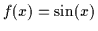(a sinusoid). This function optimization is not interesting in itself but its power will be demonstrated later when we consider arbitrary summations of different sinusoids (i.e. as in a Fourier decomposition). We derive an algorithm for optimizing an arbitrary such decomposition to yield the maximum of any landscape described by sinusoids. The extension to multiple dimensions is also discussed.

We wish to bound the single sinusoid(i.e. a one dimensional function) with a parabola as in Equation 6.17. We shall satisfy the 3 requirements (contact, tangentiality and tightness) to derive the optimization algorithm.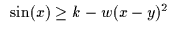(6.17)

Next consider the contact point x*=z where the parabola and the sinusoid actually touch in Equation 6.18.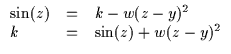(6.18)

Subsequently, we also enforce the tangentiality requirement that the derivatives are equal at contact to avoid crossover (see Equation 6.19.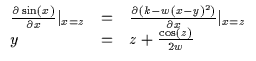(6.19)

Inserting the results of Equation 6.19 and Equation 6.18 into the inequality in Equation 6.17 we get an expression for the shape parameter,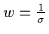as in Equation 6.20. The equation is manipulated to isolate w onto one side of the inequality. Any w can be selected now, so long as it satisfies this inequality for the given z and for all x in the domain of the function. It is subsequently straightforward to algebraically compute y and k and get a valid parabolic bound on the sinusoid for the given contact point z.

Thus, the critical step still remains: how do we compute w? A few ad hoc ways of finding such a w directly exist although these do not give an optimal value of w. Recall, now, that we also have the tightness requirement in Equation 6.7 which seeks to compute the smallest |w| or largest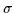. Since z is given, we wish to compute the smallest possible w that will satisfy this inequality in Equation 6.20 for all x in the domain of the function. Ultimately, we wish to recover wmin(z) which is the minimum value w can have for a given z before violating the inequality (crossing our function f(x) in some locations,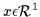and invalidating the bound).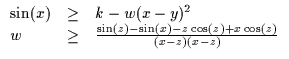(6.20)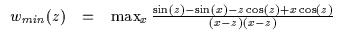(6.21)

It is difficult to find the function wmin(z) analytically for a sinusoid. Thus, for each z, we solve for wmin numerically by searching for the maximum of the right hand side expression in Equation 6.21 over all x (an efficient 1D Brent's search method is used ). The function is then stored as a lookup table (LUT) and is plotted in Figure 6.6 for visualization.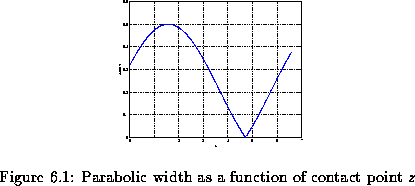Given a sinusoid f(x)=sin(x) and a contact point z, we can immediately compute wmin from this LUT and then the corresponding y (which only depends on z and w) and finally the k. A few examples of the bounding are shown in Figure 6.7.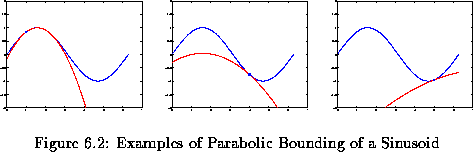Evidently, maximizing these parabolas is trivial (the maximum is y) and if z is set to y, we can repeat the calculation for a new contact point and converge to the local maximum as shown in Figure 6.8 (in fact, in this case, we converge after only one iteration).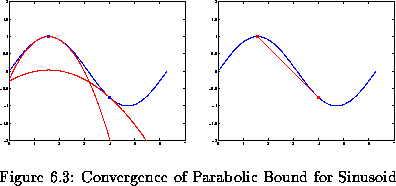At this point, optimizing the sinusoid is by no means challenging and the answer could have been solved for analytically without any of the above. However, since we have solved for the bound as opposed to a direct solution, the properties outlined earlier allow us to reapply the above derivations and solve a broader class of problems.Next: Fourier Decomposition Optimization Up: Optimization - General Bound Previous: General Bound Maximization and
Tony Jebara
1999-09-15